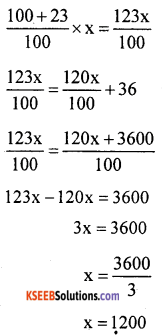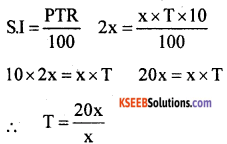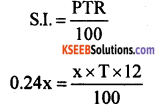# KSEEB Solutions for Class 8 Maths Chapter 9 Commercial Arithmetic Additional Questions

Students can Download Maths Chapter 9 Commercial Arithmetic Additional Questions and Answers, Notes Pdf, KSEEB Solutions for Class 8 Maths helps you to revise the complete Karnataka State Board Syllabus and score more marks in your examinations.

## Karnataka State Syllabus Class 8 Maths Chapter 9 Commercial Arithmetic Additional Questions

Question 1.
Four alternative options are given for each of the following statements. Select the correct option.
(a) Nine per cent of Rs. 700 is
A. Rs. 63
B. Rs. 630
C. Rs. 6.3
D. Rs. 0.63
Solution:
A. Rs. 63

(b) What per cent of 50 meters is 12 meters?
A. 20%
B. 60%
C. 24%
D. 32%
Solution:
C. 24%

(c) The number of whose 8% is 12 is
A. 12
B. 150
C. 130
D. 140
Solution:
B. 150

(d) An article costing Rs. 600 is sold for Rs. 750. The gain percent is
A. 20
B. 25
C. 30
D. 35
Solution:
B. 25(e) By selling a notebook for Rs. 22 a shopkeeper gains 10%. The cost price of the book is
A. Rs. 18
B. Rs. 30
C. Rs. 20
D. Rs. 22
Solution:
C. Rs. 20

(f) The percentage of loss, when an article worth Rs. 10,000 was sold for Rs. 9000 is
A. 10
B. 20
C. 15
D. 25
Solution:
A. 10

(g) A radio marked Rs. 1000 is given away for Rs. 850. The discount is
A. Rs. 50
B. Rs. 100
C. Rs. 150
D. Rs. 200
Solution:
C. Rs. 150

(h) A bookmarked Rs. 250 was sold for Rs. 200 after discount. The percentage of discount is
A. 10
B. 30
C. 20
D. 25
Solution:
C. 20

(i) The marked price of an article is 2. Rs. 200. If 15% of discount is allowed on it its selling price is
A. Rs. 185
B. Rs. 170
C. Rs. 215
D. Rs. 175
Solution:
B. Rs. 170

(j) One sells his bike through a broker by paying Rs. 200 brokerage. The rate of brokerage is 2%. The selling price of the bike is
A. Rs. 12,000
B. Rs. 10,000
C. Rs. 14,000
D. Rs. 12,500
Solution:
B. Rs. 10,000

(k) The brokerage amount for a deal is Rs. 25,000 at 2% rate of commission is
A. Rs. 500
B. Rs. 250
C. Rs. 5000
D. Rs. 2500
Solution:
A. Rs. 500

(l) If Rs. 1,600 is the commission at 8% for goods sold through a broker the selling price of the goods is
A. Rs. 18,000
B. Rs. 20,000
C. Rs. 22,000
D. Rs. 24,000
Sol:
B. Rs. 20,000

(m) The simple interest on Rs. 5000 at 2% per month for 3 months is
A. Rs. 100
B. Rs. 200
C. Rs. 300
D. Rs. 400
Solution:
C. Rs. 300(n) The time in which simple interest on a certain sum be 0.15 times the principal at 10% per annum is
A. 1.5 years
B. 1 year
C. 2 years
D. 2.5 years
Solution:
A. 1.5 years

(o) The principal that yields a simple 4. interest of Rs. 1280 at 16% per annum for 8 months is
A. Rs. 10,000
B. Rs. 12,000
C. Rs. 12,800
D. Rs. 14,000
Solution:
B. Rs. 12,000

Question 2.
A time interval of 3 minutes and 20 seconds is wrongly measured as 3 minutes and 25 seconds what is the percentage error.
Solution:
3 min 20 seconds = 180 + 20 = 200 seconds.
Difference in measurement = 205 – 200 = 5 seconds
For 200 seconds the difference in 5 seconds
For 100 seconds the difference in
$$\frac{5}{200}$$ × 100 = 2.5
∴ The percentage error is 2.5%Question 3.
Hari reads 22% of the pages of a book on the first day, 53% on the second day and 15% on the third day. If the number of pages remaining to be read is 30 find the total number of pages in the book.
Solution:
The percentage of the pages read = 22 + 53 + 15 = 90%
The percentage of the pages to be read = 100 – 90 = 10%
Let the number of pages in the book be ‘x’
Given 10% of ‘x’ is 30
$$\frac{10}{100}$$ × x = 30
$$\frac{10}{100}$$ = 30 x = 30 × 10 = 300
∴ The number of pages in the book is 300

Question 4.
If 55% of students in a school are girls and the number of boys is 270 find the number of girls in the school.
Solution:
Percentage of boys = (100 – 55) = 45%
No. of boys in the school is 270 Let the no. of students in the class be ‘x’
45% of x is 270
$$\frac{45}{100}$$ × x = 270
x = $$\frac{270 \times 100}{45}$$ = 600
No. of students = 600
∴ no. of girls = 600 – 270 = 330Question 5.
By selling an article for Rs. 920 a shopkeeper gains 15%. Find the cost price of the article.
Solution:
S.P. = Rs. 920, gain % = 15%, C.P. = ?Question 6.
Amit sells a watch at a 20% gain. Had he sold it for Rs. 36 more, he would have gained 23%. Find the cost price of the watch.
Solution:
Let the C.P. be Rs. x, gain %
= 20%Had he sold it for Rs. 36 more the new
S.P. would be Rs. $$\frac{120 x}{100}$$ + 36.
This is equal to the S.P. when the gain percent is 23%.
∴ S.P., when gain percent is 23%, is∴ The C.P. of the watch is Rs. 1200Question 7.
On selling apples at Rs. 40 per kg, a vendor incurs 10% loss. If he incurs a total loss of Rs. 120 calculate the quantity (in kg) of apples he sold.
Solution:
Loss = Rs 120, Loss % = 10%. S.P. = ?
Let the C.P. be 100 then S.P =, (100 – 10) = Rs. 90
If the loss Rs. 10 then S.P. is Rs. 90
If the loss Rs. 120 then S.P. = $$\frac{90 \times 120}{10}$$
= Rs. 1080
The total selling price
Let the quantity (in kg) of apples be ‘x’. The SP of 1 kg = Rs. 40. The S.P. of x kg = 40 x.
∴ 40x = 1080
x = $$\frac{1080}{40}$$ = 27
He sold 27 kg of apples.

Question 8.
A dealer allows a discount of 20% and still gains 20%. Find the marked price of an article that costs the dealer Rs. 720.
Solution:
C.P. = Rs. 720, gain % = 20% discount % = 20%
Gain on Rs. 720 = $$\frac{20}{100}$$ × 720 = Rs. 144
∴ S.P of the article = C.R + gain = 720 + 144 = Rs. 864
Discount percent = 20%
Let the M.R be 100 then the S.R is 100 – 20 = 80
If the S.P is Rs. 80 then M.P is Rs. 100
If the S.P is Rs. 864 the M.P is $$\frac{100 \times 864}{80}$$ = Rs. 1080
The marked price of the article is Rs. 1080Question 9.
A shopkeeper buys an article for Rs. . 600 and marks 25% above the cost price. Find (i) the selling price if he sells the article at 10% discount (ii) the percentage of discount if it is sold for Rs. 600.
Solution:
C.P = Rs. 600 and Discount rate is 25%
∴ S.P = C.P + Rate of discount × C.P.
= 600 + 25% × 600
= 600 + $$\frac{25}{100}$$ × 600
= 600 + 150 = Rs. 750
Marked price = Rs. 750

(i) Rate of discount = 10%, M.P. = Rs. 750
S.P = M.P. – discount
= 750 – $$\frac{10}{100}$$ × 750
= 750- 75
= Rs. 675

(ii) S.P = Rs. 690, M.P = 750
Discount = M.P – S.P.
= 750 – 690 = Rs. 60
If the M.P. is Rs. 750 the discount is Rs. 60. If the M.P. is 100 the discount is
$$\frac{60 \times 100}{750}$$ = 8%

Question 10.
A retailer purchases goods worth Rs. 33,600 and gets a discount of 14% from a wholesaler. For paying cash, the wholesaler gives an additional discount of 1.5% on the amount to be paid after the first discount. What is the net amount the retailer has to pay?
Solution:
M.P. = Rs. 33,600, Discount rate = 14%
Discount = $$\frac{14}{100}$$ × 33,600 = 4704
S.P = M.P – discount
= 33600 – 4704
S.P. = 28,896
S.P. after 14% discount is Rs. 28,896. He gets an additional discount of 1.5% on Rs. 28,896
Discount = $$\frac{1.5}{100}$$ × 28896
= 1.5 × 288.96
= 433.44
∴ S.P = 28896 – 433.44 = 28,462.56
The retailer has to pay Rs. 28,462.56Question 11.
An old car was disposed of through a broker for Rs. 42,000. If the brokerage is 2$$\frac{1}{2}$$ % find the amount the owner gets.
Solution:
S.P = Rs. 42,000, commission rate
= 2$$\frac{1}{2}$$ %
Commission = $$\frac{2.5}{100}$$ × 42000 – 1050
= Rs. 1050
The owner gets = 42000 – 1050 = Rs. 40,950

Question 12.
A milk-man sells 20 liters of milk every day at Rs. 22. He receives a commission of 4% every litre. Find the total commission he receives in a month of 30 days.
Solution:
The quantity of milk sold in a month of 30 days = 30 × 20 = 600
The cost of 1 liter = Rs. 22
The cost of 600 litres = 22 x 600 = Rs. 13,200
Commission rate = 4%
Commission = $$\frac{4}{100}$$ × 13200 = 528
The commission received by him in Rs. 528.

Question 13.
A bike was sold for Rs. 48,000 and a commission of Rs. 8640 was received by the dealer. Find the rate of commission.
Solution:
S.P = 48,00, commission = Rs. 8640
If the S.P. is 48,000 the commission is Rs. 8640
If the SP is 100 the commission is $$\frac{8640 \times 100}{48000}$$ = 1.8%
Rate of commission = 1.8%

Question 14.
In how many years will a sum of money becomes three times at the rate of interest 10% per annum?
Solution:
Let the principal be x. It amounts to 3x.
Simple interest = Amount – principal = 3x – x
Simple interest = 2xT = 20 years It takes 20 years.Question 15.
In what time will the simple interest on a certain sum be 0.24 times the principal at 12% per annum?
Solution:
Let the principal be ‘x’. If the simple interest is 0.24 times the principal then. simple interest = 0.24 x.100 × 0.24x = 12Tx
24x = 12Tx
∴ T = $$\frac{24 x}{12 x}$$
T = 2 years
In 2 years the simple interest will be 0.24 time the principal.

Question 16.
Find the amount of Rs. 30,000 from 15th January 2010 to 10th August 2010 at 12% per annum.
Solution:
P = Rs. 30,000, R = 12%,
T = 15th Jan 2010 to 10th Aug 2010
= 17 + 28 + 31 + 30 + 31 + 30 + 31 + 10 = 208 daysAmount = Principal + interest = 30000 + 2051.50
= Rs. 32051.50Question 17.
A person purchases electronic items worth Rs. 2,50,000. The shop keeper charges him a sales tax of 21% instead of 12%. The purchaser does not realize that he has overpaid. But after some time he finds that he has paid excess and asks the shopkeeper to return the excess ‘ money. The shopkeeper refuses and the purchaser moves the consumer court. The court with due learning orders the shop-keeper to pay the purchaser the excess money paid by the way of sales tax with an interest of 12% per annum. If the whole deliberation takes 8 months what is the money that the purchaser gets back?
Solution:
S.P = 2,50,000
Tax percent = 21%
Tax at the rate of 21%
= $$\frac{21}{100}$$ × 2,50,000 = 52,500
Rs. 52500
Tax at the rate of 12%
= $$\frac{12}{100}$$ × 2,50,000 = 30,000 100
Rs. 30,000
Excess paid = 52,500 – 30,000
= Rs. 22,500
Now P = 22,500, R = 12%,
T = 8 months = $$\frac{8}{12}$$ yearsAmount = Principal + Interest = 22,500 + 1800 = 24,300
The purchaser gets back Rs. 24,300.error: Content is protected !!